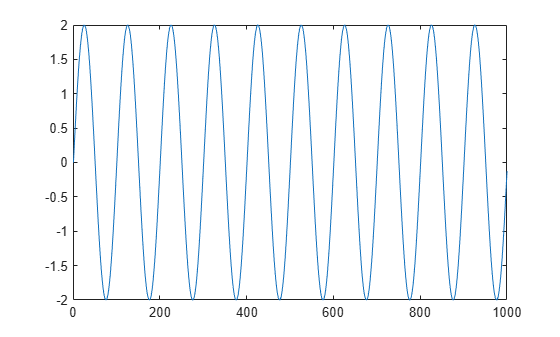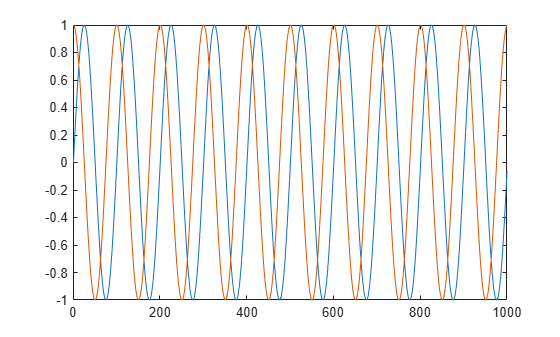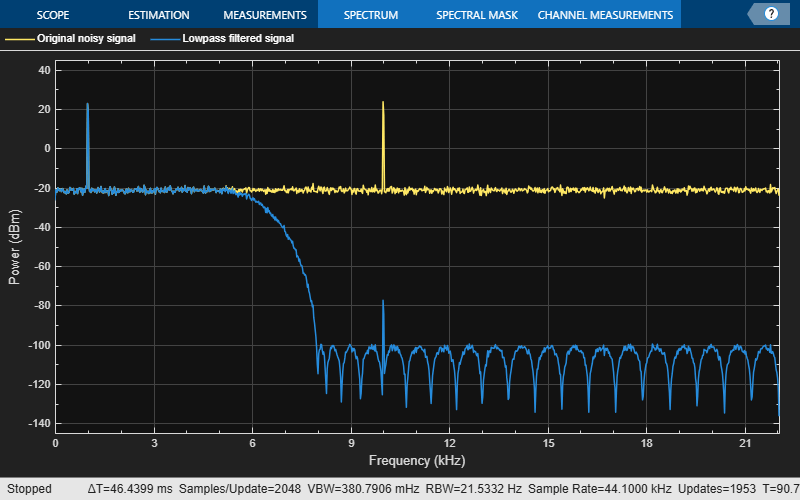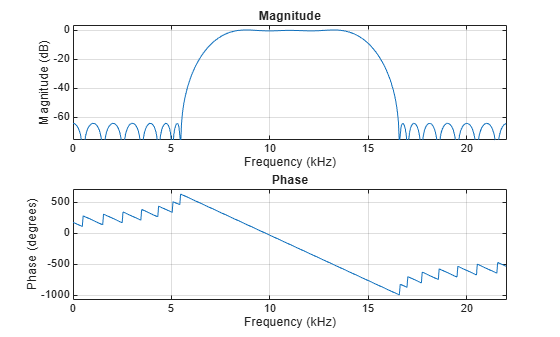# dsp.SineWave

Generate discrete sine wave

## Description

The `dsp.SineWave` System object™ generates a real or complex, multichannel sinusoidal signal with independent amplitude, frequency, and phase in each output channel.

For both real and complex sinusoids, the Amplitude, Frequency, and PhaseOffset properties can be scalars or length-N vectors, where N is the number of channels in the output. When you specify at least one of these properties as a length-N vector, scalar values specified for the other properties are applied to each of the N channels.

To generate a discrete-time sinusoidal signal:

1. Create the `dsp.SineWave` object and set its properties.

2. Call the object with arguments, as if it were a function.

## Creation

### Syntax

``sine = dsp.SineWave``
``sine = dsp.SineWave(Name,Value)``
``sine = dsp.SineWave(amp,freq,phase,Name,Value)``

### Description

````sine = dsp.SineWave` creates a sine wave object that generates a real-valued sinusoid with an amplitude of 1, a frequency of 100 Hz, and a phase offset of 0. By default, the sine wave object generates only one sample.```
````sine = dsp.SineWave(Name,Value)` creates a sine wave object with each specified property set to the specified value. Enclose each property name in single quotes. Example: sine = dsp.SineWave('Amplitude',2);```

example

````sine = dsp.SineWave(amp,freq,phase,Name,Value)` creates a sine wave object with the Amplitude property set to `amp`, Frequency property set to `freq`, PhaseOffset property set to `phase`, and anyother specified properties set to the specified values.```

## Properties

expand all

Unless otherwise indicated, properties are nontunable, which means you cannot change their values after calling the object. Objects lock when you call them, and the `release` function unlocks them.

If a property is tunable, you can change its value at any time.

Amplitude of the sine wave, specified as one of the following:

• scalar –– A scalar applies to all channels.

• vector –– A length-N vector contains the amplitudes of the sine waves in each of the N output channels. The vector length must be the same as that specified for the Frequency and PhaseOffset properties.

Tunable: Yes

#### Dependencies

This property is tunable only when you set Method to either ```'Trigonometric function'``` or `'Differential'`.

Data Types: `single` | `double` | `int8` | `int16` | `int32` | `int64` | `uint8` | `uint16` | `uint32` | `uint64`

Frequency of the sine wave in Hz, specified as one of the following:

• scalar –– A scalar applies to all channels.

• vector –– A length-N vector contains the frequencies of the sine waves in each of the N output channels. The vector length must be the same as that specified for the Amplitude and PhaseOffset properties.

Data Types: `single` | `double` | `int8` | `int16` | `int32` | `int64` | `uint8` | `uint16` | `uint32` | `uint64`

Phase offset of the sine wave in radians, specified as one of the following:

• scalar –– A scalar applies to all channels.

• vector –– A length-N vector contains the phase offsets of the sine waves in each of the N output channels. The vector length must be the same as that specified for the Amplitude and Frequency properties.

Data Types: `single` | `double` | `int8` | `int16` | `int32` | `int64` | `uint8` | `uint16` | `uint32` | `uint64`

Flag that indicates whether the waveform is real or complex, specified as either:

• `false` –– The waveform output is real.

• `true` –– The waveform output is complex.

Method used to generate sinusoids, specified as one of the following:

• `'Trigonometric function'` –– The object computes the sinusoid by sampling the continuous-time function.

• `'Table lookup'` –– The object precomputes the unique samples of every output sinusoid at the start of the simulation, and recalls the samples from memory as needed.

• `'Differential'` –– The object uses an incremental algorithm. This algorithm computes the output samples based on the output values computed at the previous sample time and precomputed update terms.

Optimize table of sine values for speed or memory, specified as either:

• `'Speed'` –– The table contains k elements, where k is the number of input samples in one full period of the sine wave. The period of each sinusoid must be an integer multiple of 1/Fs, where Fs is the value of the SampleRate property value. That is, each element of the Frequency property must be of the form Fs/m, where m is an integer greater than `1`.

• `'Memory'` –– The table contains k/4 elements.

#### Dependencies

This property applies only when you set the `Method` property to `'Table lookup'`.

Sample rate of output signal in Hz, specified as a positive scalar.

Example: `44100`

Example: `22050`

Number of consecutive samples from each sinusoid to buffer into the output frame, specified as a positive integer.

Example: `1000`

Example: `5000`

Data Types: `single` | `double` | `int8` | `int16` | `int32` | `int64` | `uint8` | `uint16` | `uint32` | `uint64`

Data type of the sine wave output, specified as `'double'`, `'single'`, or `'Custom'`.

Fixed-Point Properties

Output word and fraction lengths, specified as an autosigned numeric type with a word length of 16.

Example: numerictype([],32,30)

Example: numerictype([],16,15)

#### Dependencies

This property applies only when you set the Method property to ```'Table lookup'``` and the OutputDataType property to `'Custom'`.

## Usage

### Syntax

``sineOut = sine()``

### Description

example

````sineOut = sine()` creates the sine wave output, `sineOut`.```

### Output Arguments

expand all

Sine wave output, returned as a vector or matrix. The SamplesPerFrame property determines the number of rows in the output matrix. If the Frequency or the PhaseOffset property is a vector, the length of the vector determines the number of columns (channels) in the output matrix. If the `Frequency` or the `PhaseOffset` properties is a scalar, then the number of channels in the output matrix is 1.

The OutputDataType property sets the data type of the output.

Data Types: `single` | `double` | `fi`

## Object Functions

To use an object function, specify the System object as the first input argument. For example, to release system resources of a System object named `obj`, use this syntax:

`release(obj)`

expand all

 `step` Run System object algorithm `release` Release resources and allow changes to System object property values and input characteristics `reset` Reset internal states of System object

## Examples

collapse all

Note: If you are using R2016a or an earlier release, replace each call to the object with the equivalent `step `syntax. For example, `obj(x) `becomes `step(obj,x)`.

Generate a sine wave with an amplitude of 2, frequency of 10 Hz, and an initial phase of 0.

```sine1 = dsp.SineWave(2,10); sine1.SamplesPerFrame = 1000; y = sine1(); plot(y)```Generate two sine waves offset by a phase of pi/2 radians.

```sine2 = dsp.SineWave; sine2.Frequency = 10; sine2.PhaseOffset = [0 pi/2]; sine2.SamplesPerFrame = 1000; y = sine2(); plot(y)```This example shows how to lowpass filter a noisy signal in MATLAB and visualize the original and filtered signals using a spectrum analyzer. For a Simulink version of this example, see Filter Frames of a Noisy Sine Wave Signal in Simulink.

Specify Signal Source

The input signal is the sum of two sine waves with frequencies of 1 kHz and 10 kHz. The sampling frequency is 44.1 kHz.

```Sine1 = dsp.SineWave('Frequency',1e3,'SampleRate',44.1e3); Sine2 = dsp.SineWave('Frequency',10e3,'SampleRate',44.1e3); ```

Create Lowpass Filter

The lowpass FIR filter, `dsp.LowpassFilter`, designs a minimum-order FIR lowpass filter using the generalized Remez FIR filter design algorithm. Set the passband frequency to 5000 Hz and the stopband frequency to 8000 Hz. The passband ripple is 0.1 dB and the stopband attenuation is 80 dB.

```FIRLowPass = dsp.LowpassFilter('PassbandFrequency',5000,... 'StopbandFrequency',8000); ```

Create Spectrum Analyzer

Set up the spectrum analyzer to compare the power spectra of the original and filtered signals. The spectrum units are dBm.

```SpecAna = dsp.SpectrumAnalyzer('PlotAsTwoSidedSpectrum',false, ... 'SampleRate',Sine1.SampleRate, ... 'NumInputPorts',2,... 'ShowLegend',true, ... 'YLimits',[-145,45]); SpecAna.ChannelNames = {'Original noisy signal','Low pass filtered signal'}; ```

Specify Samples per Frame

This example uses frame-based processing, where data is processed one frame at a time. Each frame of data contains sequential samples from an independent channel. Frame-based processing is advantageous for many signal processing applications because you can process multiple samples at once. By buffering your data into frames and processing multisample frames of data, you can improve the computational time of your signal processing algorithms. Set the number of samples per frame to 4000.

```Sine1.SamplesPerFrame = 4000; Sine2.SamplesPerFrame = 4000; ```

Filter the Noisy Sine Wave Signal

Add zero-mean white Gaussian noise with a standard deviation of 0.1 to the sum of sine waves. Filter the result using the FIR filter. While running the simulation, the spectrum analyzer shows that frequencies above 8000 Hz in the source signal are attenuated. The resulting signal maintains the peak at 1 kHz because it falls in the passband of the lowpass filter.

```for i = 1 : 1000 x = Sine1()+Sine2()+0.1.*randn(Sine1.SamplesPerFrame,1); y = FIRLowPass(x); SpecAna(x,y); end release(SpecAna) ```Bandpass filter a discrete-time sine wave signal which consists of three sinusoids at frequencies, 1 kHz, 10 kHz, and 15 kHz.

Design an FIR Equiripple bandpass filter by first creating a bandpass filter design specifications object, and then designing a filter using these specifications.

Design Bandpass Filter

Create a bandpass filter design specifications object using `fdesign.bandpass`.

```bandpassSpecs = fdesign.bandpass('Fst1,Fp1,Fp2,Fst2,Ast1,Ap,Ast2', ... 1/4,3/8,5/8,6/8,60,1,60);```

List the available design methods for this object.

`designmethods(bandpassSpecs)`
```Design Methods for class fdesign.bandpass (Fst1,Fp1,Fp2,Fst2,Ast1,Ap,Ast2): butter cheby1 cheby2 ellip equiripple kaiserwin ```

To design an Equiripple filter, pick `'equiripple'`.

`bpFilter = design(bandpassSpecs,'equiripple','Systemobject',true)`
```bpFilter = dsp.FIRFilter with properties: Structure: 'Direct form' NumeratorSource: 'Property' Numerator: [1x37 double] InitialConditions: 0 Show all properties ```

Visualize the frequency response of the designed filter.

`fvtool(bpFilter,'Fs',44100)`Create Sinusoidal Signal

Create a signal that is a sum of three sinusoids with frequencies at 1 kHz, 10 kHz, and 15 kHz. Initialize Spectrum Analyzer to view the original signal and the filtered signal.

```Sine1 = dsp.SineWave('Frequency',1e3,'SampleRate',44.1e3,'SamplesPerFrame',4000); Sine2 = dsp.SineWave('Frequency',10e3,'SampleRate',44.1e3,'SamplesPerFrame',4000); Sine3 = dsp.SineWave('Frequency',15e3,'SampleRate',44.1e3,'SamplesPerFrame',4000); SpecAna = dsp.SpectrumAnalyzer('PlotAsTwoSidedSpectrum',false, ... 'SampleRate',Sine1.SampleRate, ... 'NumInputPorts',2,... 'ShowLegend',true, ... 'YLimits',[-240,45]); SpecAna.ChannelNames = {'Original noisy signal','Bandpass filtered signal'};```

Filter Sinusoidal Signal

Filter the sinusoidal signal using the bandpass filter that has been designed. View the original signal and the filtered signal in the Spectrum Analyzer. The tone at 1 kHz is filtered out and attenuated. The tone at 10 kHz is unaffected, and the tone at 15 kHz is mildly attenuated because it appears in the transition band of the filter.

```for i = 1 : 1000 x = Sine1()+Sine2()+Sine3(); y = bpFilter(x); SpecAna(x,y); end release(SpecAna)```expand all

expand all

## Extended Capabilities

### Objects

Introduced in R2012a

## SupportGet trial now